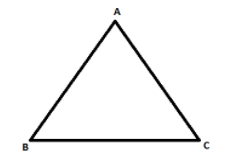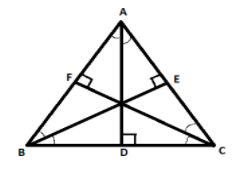QuestionAnswers

# Prove that the circumcenter, orthocenter, incenter and centroid of the triangle formed by the points A (-1, 11), B (-9, -8) and C (15,-2) are collinear, without actually finding any of them.Verified
128.4k+ views
Hint: Here in this question we will use the concept of distance formula so that we can prove that the points are collinear.
Definition of collinear points: - Three or more points are said to be collinear if they lie on a single straight line.
We will use distance formula between the two points ${x_1},{y_1}$ and ${x_2},{y_2}$ that is mentioned below: -
$d = \sqrt {{{({x_2} - {x_1})}^2} + {{({y_2} - {y_1})}^2}}$ d= distance between two points.

Draw a triangle ABC having points A (-1, 11), B (-9, -8) and C (15,-2)Points for AB are A (-1, 11) and B (-9, -8)
$\Rightarrow AB = \sqrt {{{[ - 9 + 1]}^2} + {{[ - 8 - 11]}^2}}$ (Putting values in distance formula $d = \sqrt {{{({x_2} - {x_1})}^2} + {{({y_2} - {y_1})}^2}}$ )
$\Rightarrow AB = \sqrt {{{[ - 8]}^2} + {{[ - 19]}^2}}$
$\Rightarrow AB = \sqrt {64 + 361}$
$\Rightarrow AB = \sqrt {425}$
Points for BC are B (-9, -8) and C (15, -2)
$\Rightarrow BC = \sqrt {{{[15 + 9]}^2} + {{[ - 2 + 8]}^2}}$ (Putting values in distance formula $d = \sqrt {{{({x_2} - {x_1})}^2} + {{({y_2} - {y_1})}^2}}$ )
$\Rightarrow BC = \sqrt {{{}^2} + {{}^2}}$
$\Rightarrow BC = \sqrt {576 + 36}$
$\Rightarrow BC = \sqrt {612}$
Points for AC are A (-1, 11) and C (15, -2)
$\Rightarrow AC = \sqrt {{{[15 + 1]}^2} + {{[ - 2 - 11]}^2}}$ (Putting values in distance formula $d = \sqrt {{{({x_2} - {x_1})}^2} + {{({y_2} - {y_1})}^2}}$ )
$\Rightarrow AC = \sqrt {{{}^2} + {{[ - 13]}^2}}$
$\Rightarrow AC = \sqrt {256 + 169}$
$\Rightarrow AC = \sqrt {425}$
From above we observe that side AB and AC are equal so the given points make an isosceles triangle. Isosceles triangle is that triangle whose two sides are equal and corresponding angles to those sides are also equal. Therefore $\angle ABC = \angle ACB$
Now draw perpendicular from point ‘A’ to the side BC bisecting side BC into two equal parts. As we know from the isosceles triangle property that perpendicular is same as median in isosceles triangle so therefore AD will be called as altitude, perpendicular bisector and median to the side BC.Here without solving we can clearly see that circumcenter, orthocentre, incenter and centroid all lie in the same line AD.

Note: Some students may find confusion in the definition of all these centres of the triangle so below all definitions are being mentioned for greater understanding.
*Circumcenter: - It is defined as that point where all the perpendicular bisectors of the sides of the triangle intersect.
*Orthocenter: -It is the intersection of three altitudes of a triangle.
*Incenter: -It is a point where internal angle bisectors of a triangle meet.
*Centroid: -It is the intersection of the three medians of the triangle.